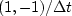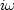Next: CAUSAL INTEGRATION RECURSION Up: DERIVATIVE AND INTEGRAL Previous: DERIVATIVE AND INTEGRAL

Given a sampled signal, its time derivative can be estimated by convolution with the filter.This could be done with any convolution program. For example if we choose to ignore end effects we might select convin(). This example arises so frequently that I display the matrix multiply below:(22)

The filter impulse response is seen in any column in the middle of the matrix, namely (1,-1). In the transposed matrix the filter impulse response is time reversed to (-1,1). So, mathematically, we can say that the adjoint of the time derivative operation is the negative time derivative. This corresponds also to the fact that the complex conjugate ofis.We can also speak of the adjoint of the boundary conditions: we might say the adjoint of no boundary condition'' is specified value'' boundary conditions.

Banded matrices like in (21) and (22) arise commonly, and subroutines like convin()are awkward and over-general because they sum with a do loop where a mere statement of the two terms is enough. This is illustrated in subroutine ruffen1(). Notice the adjoint calculation resembles that in spot1().

subroutine ruffen1( adj,    n, xx, yy     )
real                        xx(n), yy( n-1)
do i= 1, n-1 {
yy(i) = yy(i) + xx(i+1) - xx(i)
else {
xx(i+1) = xx(i+1) + yy(i)
xx(i  ) = xx(i  ) - yy(i)
}
}
return; endNext: CAUSAL INTEGRATION RECURSION Up: DERIVATIVE AND INTEGRAL Previous: DERIVATIVE AND INTEGRAL
Stanford Exploration Project
10/21/1998